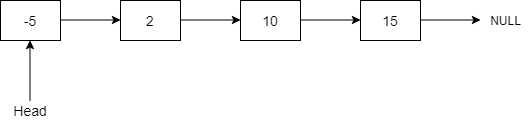# Delete Node In A Linked List

Posted: 25 Jan, 2021
Difficulty: Easy

## PROBLEM STATEMENT

#### Note:

``````• The reference to the head of the linked list is not given.
• The node to be deleted is not a tail node.
• The value of each node in the Linked List is unique.
• It is guaranteed that the node to be deleted is present in the linked list.
``````##### Input format:
``````The first line contains an integer 'T' which denotes the number of test cases or queries to be run. Then, the T test cases follow.

The first line of each test case contains space-separated integers denoting the values of nodes of the Linked List. The Linked List is terminated with -1. Hence, -1 is never a node value of the Linked List.

The second line of each test case contains a single integer K which denotes the node to be deleted from the Linked List.

For more clarity please refer to the sample inputs.
``````
##### Output format:
``````For each test case, delete the given node and then print a single line containing the elements of the Linked List separated by a single space, '-1' at the end denotes the end of the linked list.

The output for each test case will be printed in a new line.
``````
##### Note:
``````You do not need to print anything. It has already been taken care of. Just implement the given function.
``````
##### Constraints:
``````1 <= T <= 100
2 <= N <= 5000
-10 ^ 9 <= NODE.DATA <= 10 ^ 9 and node.data != -1

Where 'N' denotes the total number of nodes in the Linked List and 'NODE.DATA' is the value of the node present.

Time limit: 1 sec.
``````Approach 1

Approach:

We need to delete the node K from the linked list. The most general way to delete the node K from a singly linked list is to get access to the previous node of K. We can get access to the previous node by traversing from the head of the linked list. Let’s denote this previous node as P. Then, we update the next pointer of P to the next pointer of K

Although the reference to node K is given, we do not have access to the head of the linked list. So, there is no way in which we can reach the previous node of K. Thus we have to store the data of the next node of K in the node K itself and then delete the next node of K by making K.next = K.next.next

Steps:

1. Create a temp pointer that points to the next node of the node K i.e. temp = K.next
2. Store the data of temp in the node K and make K.next = temp.next.
3. Finally, delete/free the temp node. Note, that in languages like C/C++, we do this step to avoid memory leak.